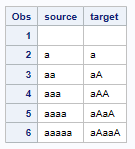## Nth letter Capitalized in a string

``````/* Using PROC SQL */
proc sql;

select
substr(Name, 1, 1) || propcase(substr(Name, 2, 1)) || substr(Name, 3) as NewVariable
from sashelp.class;
quit;``````

how to capitalized 2nd letter using array and do loop in datastep method

1 ACCEPTED SOLUTION

Accepted Solutions

## Re: Nth letter Capitalized in a string

Not sure why you would use a loop (or why you are using PROPCASE() instead of UPCASE()).

Just replace the 2 letter with its uppercase version.

``````data want;
set sashelp.class;
substr(name,2,1)=upcase(substr(name,2,1));
run;``````

The only reason to use an ARRAY would be if there were multiple variables you wanted to do the same operation on.   In that case watch out for variables that do not have room for a second letter.

``````data want;
set sashelp.class;
array c _character_;
do over c;
if vlength(c) > 1 then substr(c,2,1)=upcase(substr(c,2,1));
end;
run;``````

If you don't like using features SAS is trying to disavow (This tape will self-destruct in 5 seconds...) then introduce an index variable to the DO loop and the array references.

``````data want;
set sashelp.class;
array c _character_;
do index=1 to dim(c);
if vlength(c[index]) > 1 then substr(c[index],2,1)=upcase(substr(c[index],2,1));
end;
drop index;
run;``````

5 REPLIES 5

## Re: Nth letter Capitalized in a string

Not sure why you would use a loop (or why you are using PROPCASE() instead of UPCASE()).

Just replace the 2 letter with its uppercase version.

``````data want;
set sashelp.class;
substr(name,2,1)=upcase(substr(name,2,1));
run;``````

The only reason to use an ARRAY would be if there were multiple variables you wanted to do the same operation on.   In that case watch out for variables that do not have room for a second letter.

``````data want;
set sashelp.class;
array c _character_;
do over c;
if vlength(c) > 1 then substr(c,2,1)=upcase(substr(c,2,1));
end;
run;``````

If you don't like using features SAS is trying to disavow (This tape will self-destruct in 5 seconds...) then introduce an index variable to the DO loop and the array references.

``````data want;
set sashelp.class;
array c _character_;
do index=1 to dim(c);
if vlength(c[index]) > 1 then substr(c[index],2,1)=upcase(substr(c[index],2,1));
end;
drop index;
run;``````

## Re: Nth letter Capitalized in a string

Hi Tom,

Thank you very much your different solutions

suppose i want to capitalized 2nd and last letters how can solve  substr not working for last letter

``````data;
set sashelp.class;
substr(name,2,1)=upcase(substr(name,2,1));
substr(name,-1,1)=upcase(substr(name,-1,1));
run;``````

## Re: Nth letter Capitalized in a string

SUBSTR() works fine for the last character.  You just can't used negative positions as that has no meaning.

``substr(name,length(name),1)=upcase(substr(name,length(name),1));``

## Re: Nth letter Capitalized in a string

``````data fl;
set sashelp.class;
substr(name,2,1)=upcase(substr(name,2,1));
substr(name,length(name),1)=upcase(substr(name,length(name),1));
proc print noobs;
run;``````

Thanks Tom

## Re: Nth letter Capitalized in a string

Just for fun adding a RegEx option. It also covers your later requirement to uppercase the last character in the string.

``````data test;
length source target \$5;
do source=' ','a','aa','aaa','aaaa','aaaaa';
target=prxchange('s/^(.)(.)(.*?)(.?)\$/\$1\u\$2\$3\u\$4/oi',1,strip(source));
output;
end;
run;
proc print data=test;
run;
``````Discussion stats
• 5 replies
• 415 views
• 1 like
• 3 in conversation